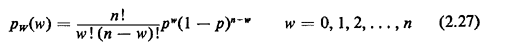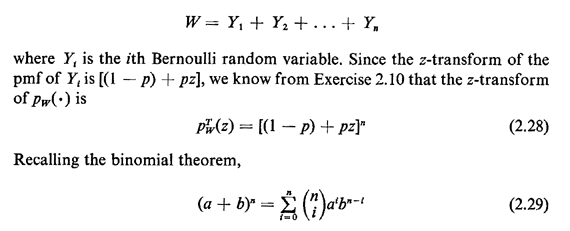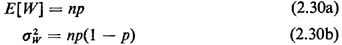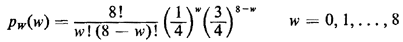## 2.9.3 Binomial PMF

A random variable W has a binomial pmf ifHere W can be interpreted to be the number of successes in n independent Bernoulli trials, each having success probability p. We can see this by writingand the expanded form of the z-transform, (2.14), we obtain the binomial pmf shown in (2-27). By considering W to be the sum of n independent, identically distributed Bernoulli random variables, we obtain the mean and variance by inspection:In the police patrol example, with p = 0.25, the probability that the high-crime zone receives exactly w hours of patrol during an 8-hour tour of duty is